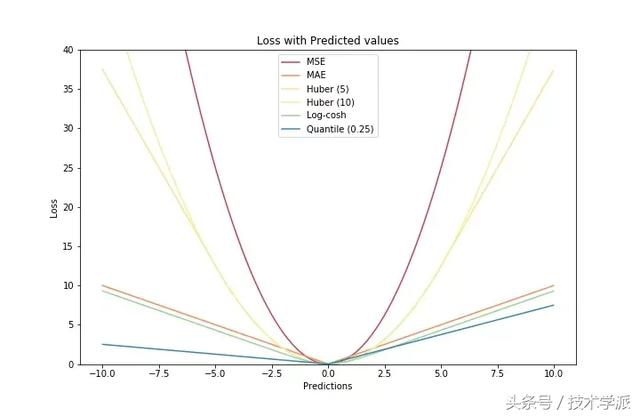# 入门机器学习必备！五种回归损失函数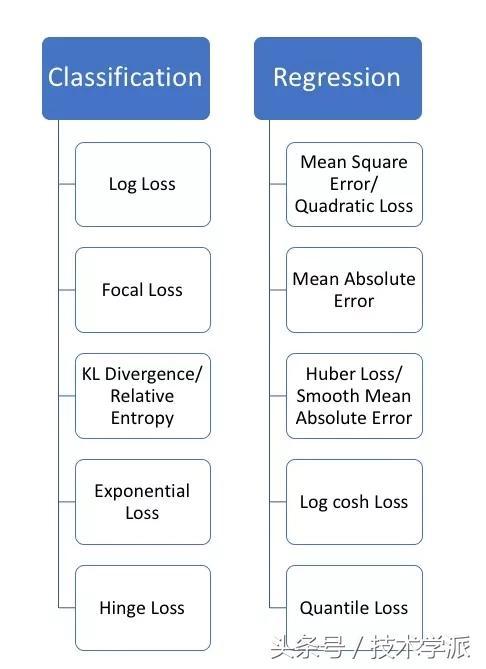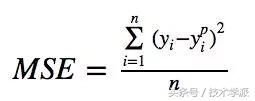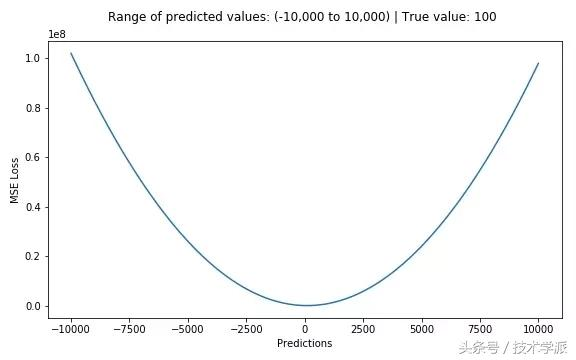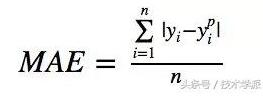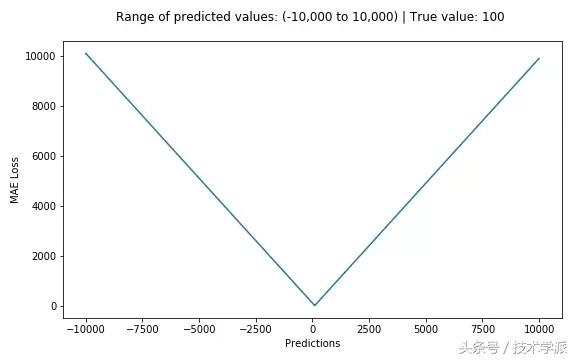L2损失 vs L1损失

# true: 真目标变量的数组

# pred: 预测值的数组

def mse(true, pred):

return np.sum((true - pred)**2)

def mae(true, pred):

return np.sum(np.abs(true - pred))

# 在 sklearn 中同样适用

from sklearn.metrics import mean_squared_error

from sklearn.metrics import mean_absolute_error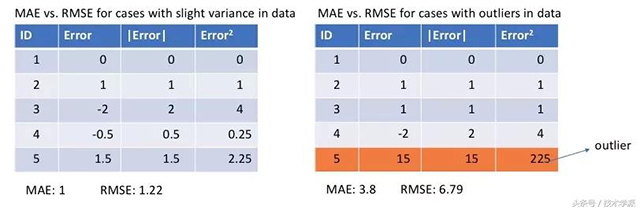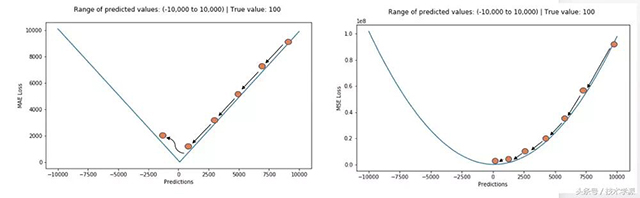L1损失对异常值更鲁棒，但它的导数是不连续的，从而让它无法有效的求解。L2损失对异常值很敏感，但会求出更稳定和更接近的解（通过将导数设为0）。

Huber损失函数，平滑平均绝对误差 相比平方误差损失，Huber损失对于数据中异常值的敏感性要差一些。在值为0时，它也是可微分的。它基本上是绝对值，在误差很小时会变为平方值。误差使其平方值的大小如何取决于一个超参数δ，该参数可以调整。当δ~ 0时，Huber损失会趋向于MAE；当δ~ ∞（很大的数字），Huber损失会趋向于MSE。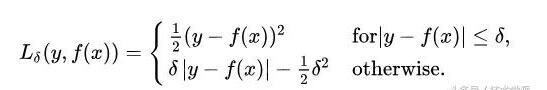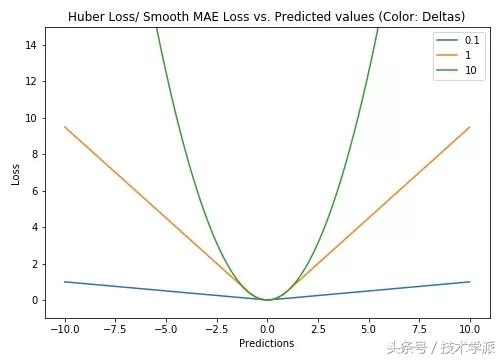δ的选择非常关键，因为它决定了你如何看待异常值。残差大于δ，就用L1（它对很大的异常值敏感性较差）最小化，而残差小于δ，就用L2“适当地”最小化。

Log-Cosh损失函数

Log-Cosh是应用于回归任务中的另一种损失函数，它比L2损失更平滑。Log-cosh是预测误差的双曲余弦的对数。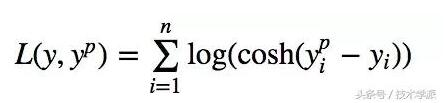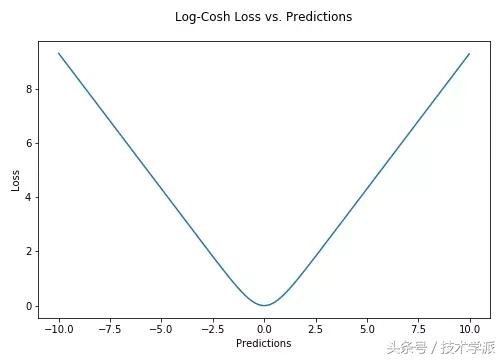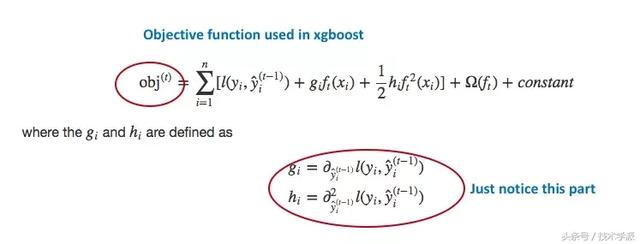Huber损失函数和Log-cosh损失函数的Python代码：

# huber 损失

def huber(true, pred, delta):

loss = np.where(np.abs(true-pred) < delta , 0.5*((true-pred)**2), delta*np.abs(true - pred) - 0.5*(delta**2))

return np.sum(loss)

?

# log cosh 损失

def logcosh(true, pred):

loss = np.log(np.cosh(pred - true))

return np.sum(loss)

Quantile损失函数 在大多数现实预测问题中，我们常常很想知道我们的预测值的不确定性。对于很多业务问题而言，相对于知道某个预测点，了解预测值范围能够大幅优化决策过程。最小二乘回归的预测区间基于我们假设残差值（y?—?y_hat）在所有独立变量值上的变化保持一致。

Quantile回归 VS 普通最小乘二回归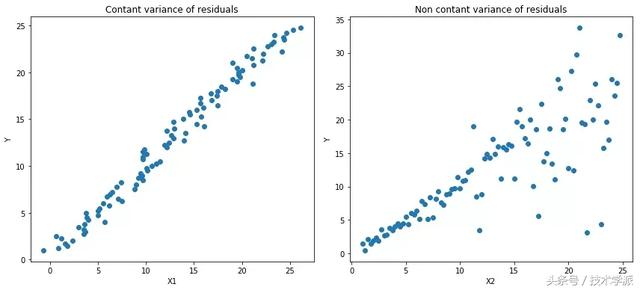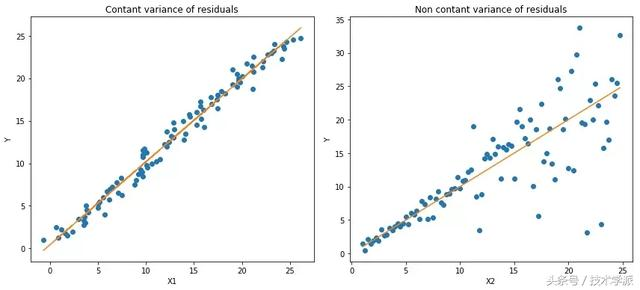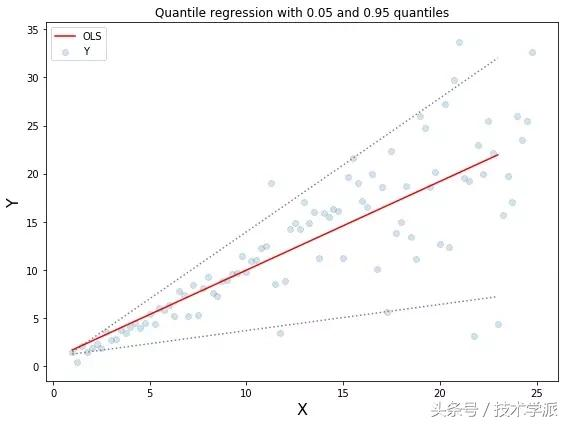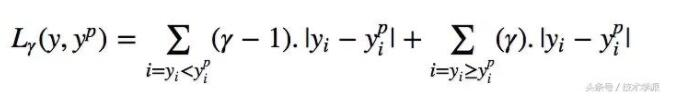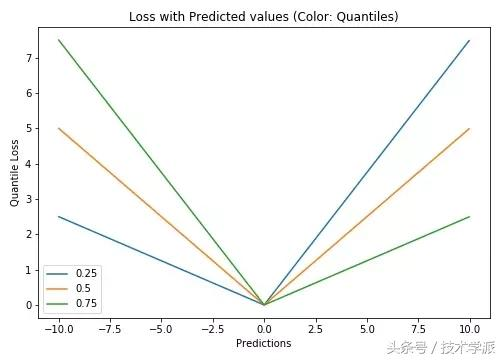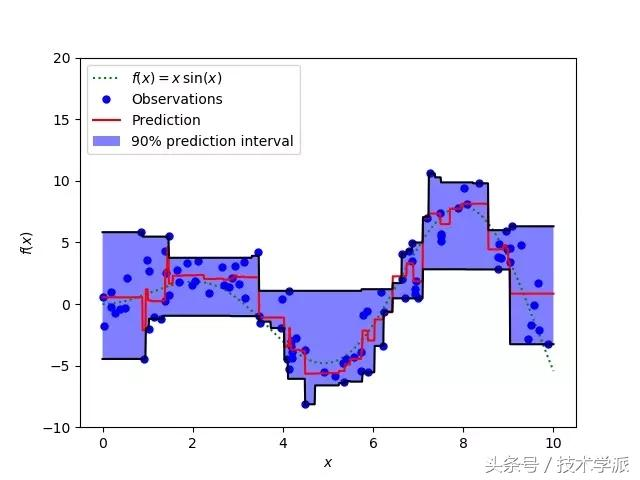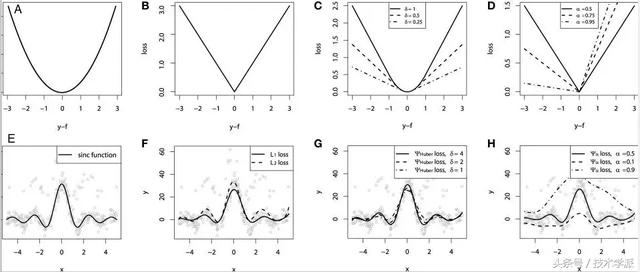Quantile损失在对应置信度水平上做出了很好的预测。